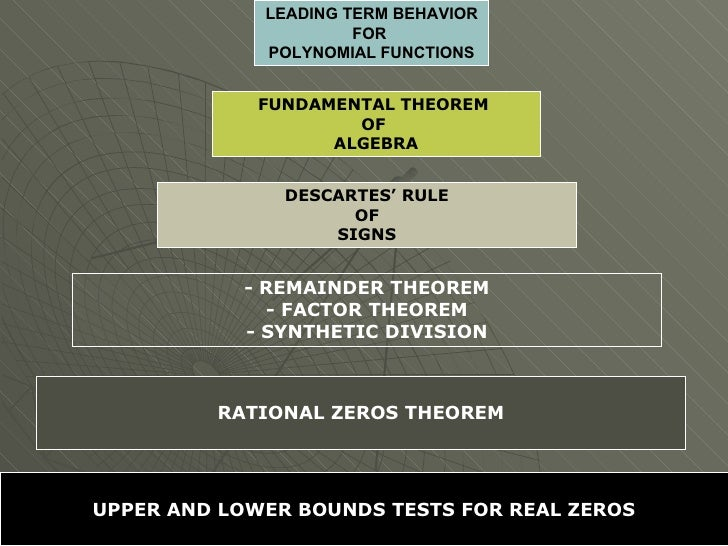Successfully reported this slideshow.Upcoming SlideShare
×

# Making Connections

2,969 views

Published on

This PowerPoint is for my PreCalculus class and is used to tie together the major concepts from Chapter 2: Polynomials of Higher Degree

• Full Name
Comment goes here.

Are you sure you want to Yes No• Be the first to comment

### Making Connections

1. 1. LEADING TERM BEHAVIOR FOR POLYNOMIAL FUNCTIONS FUNDAMENTAL THEOREM OF ALGEBRA DESCARTES’ RULE OF SIGNS - REMAINDER THEOREM - FACTOR THEOREM - SYNTHETIC DIVISION RATIONAL ZEROS THEOREM UPPER AND LOWER BOUNDS TESTS FOR REAL ZEROS
2. 2. HOME
3. 3. FUNDAMENTAL THEOREM OF ALGEBRA <ul><li>A polynomial function of degree n > 0 has n complex zeros. Some of these zeros may be repeated. </li></ul>HOME GO TO:http://www.wmueller.com/precalculus/families/1_51.html
4. 4. DESCARTES’ RULE OF SIGNS A polynomial has no more positive roots than it has sign changes. The idea of a sign change is a simple one. Consider the polynomial: Proceeding from left to right, we see that the terms of the polynomial carry the signs + - + - for a total of 3 sign changes. Descartes’ Rule of Signs tells us that this polynomial may have up to three positive roots. P(x) = x 3 – 8x 2 + 17x - 10 NEXT
5. 5. A polynomial has no more negative roots than P(-x) has sign changes. To use this, we just , we just replace every instance of x with –x in our polynomial. This essentially changes the signs of the odd powers but not the even ones. Thus: P(-x) = -x 3 – 8x 2 - 17x - 10 P(x) = x 3 – 8x 2 + 17x - 10 P(-x) has no sign changes and so P(x) has no negative roots. NEXT BACK
6. 6. Just as the Fundamental Theorem of Algebra gives us an upper bound on the total number of roots of a polynomial, Descartes’ Rule of Signs gives us an upper bound on the total number of positive ones. A polynomial may not achieve the maximum allowable number of roots given by the Fundamental Theorem, and likewise it may not achieve the maximum allowable number of positive roots given by the Rule of Signs. NEXT BACK
7. 7. g(x) = x 3 – x 2 + 1 g(-x) = -x 3 – x 2 + 1 g(-x) has one sign change and, so at most it has one negative root HOME
8. 8. REMAINDER THEOREM: If a polynomial f(x) is divided by x – k, then the remainder is r = f(k) FACTOR THEOREM: If a polynomial function f(x) has a factor x – k if and only if f(k) = 0 SYNTHETIC DIVISION: Use to find both factor and remainder of a function NEXT
9. 9. For example: When f(x) = 3x 2 + 7x - 20 is divided by x + 4 using synthetic division we see that there is a remainder of zero. So by the Factor Theorem, x + 4 is a factor of f(x) because there is a remainder of 0. In other words, we can say: NEXT
10. 10. If we used the Remainder Theorem….. we would find that f(-4) = 0 Since we have a remainder of 0, x + 4 must be a factor HOME f(x) 3x 2 + 7x - 20 f(-4) = 3(-4) 2 + 7(-4) - 20 f(-4) = 3(16) - 28 - 20 f(-4) = 48 - 28 - 20 f(-4) = 0
11. 11. RATIONAL ZEROS THEOREM The RATIONAL ZEROS THEOREM is a way to limit the possible number of candidates tested with either synthetic division or the remainder theorem to find rational zeros of the polynomial. For example: f(x) = x 3 – 3x 2 + 1 Because the leading and constant coefficients are both 1, according to the Rational Zeros Theorem, the only potential rational zeros of f are 1 and -1. So we check to see if they are in fact zeros of f: f(1) = (1) 3 – 3(1) 2 + 1 = 1 since f(1) does not equal zero, f(x) has no rational zeros f(-1) = (-1) 3 – 3(-1) 2 + 1 = -3 since f(-1) does not equal zero, f(x) has no rational zeros HOME
12. 12. UPPER AND LOWER BOUNDS TESTS FOR REAL ZEROS The Upper and Lower Bounds Tests for Real Zeros is used to confirm that all real zeros lie on a given interval. HOME